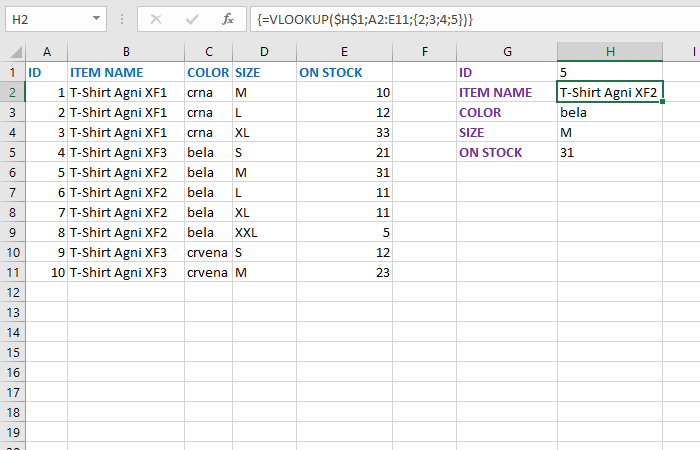# Constants

Few Excel users know about array formulas and I believe they have found themselves in a situation where they need them at least once. They were mentioned in one of the previous “recipes”, and here you will be able to learn how to use them in combination with constants. Constants are values ​​inside curly braces, separated by a comma (or semicolon), and we use them for arithmetic operations within an array formula.

Let’s start with a simple table that shows: id, item name, color, size and stock value. If we need to find, for example, a color based on the id, we will use the VLOOKUP formula in which we refer to the column in which the color names are displayed:

=VLOOKUP(\$H\$1,A2:E11,3)

If we wanted to find the size the last argument would be the number 4 etc. Is it possible to write one formula to find all the required attributes of the item? Yes, and in that case we create an array formula, and as the last argument of the VLOOKUP function we will use constants – values ​​listed in order and framed by curly brackets.

If in column G we state the values: ID, ITEM NAME, COLOR, SIZE and ON STOCK, and in H1 we enter an arbitrary ID, in the remaining cells we need to enter the formula:

=VLOOKUP(\$H\$1,A2:E11,{2,3,4,5})

All four cells must be selected before input. Finish the entry, as with all array formulas, by pressing CTRL + SHIFT + ENTER. Excel will automatically find the values ​​of the remaining columns and display them in a table.